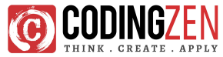## Introduction

Python is and has been the go-to language for machine learning for years, possibly due to its easy-to-learn syntax with powerful Autograd modules. In addition, these modules themselves translate and execute the code in C and are very fast.

AutoGrad stands for Automatic Gradient, and Gradient is the key part of the best Classification algorithms in Machine Learning. Linear Regression/ Logistic Regression and Deep Convolutional Neural Networks are algorithms that benefit from this Automatic gradient, which keeps track of every operation performed.

The network we will use today to achieve our goal is a Convolution Neural Network, specially made to handle image Data.

By the end, we expect to create a program that can tell the difference between a Male and a Female from an image.

Tech Stack

Jupyter Notebook: We will write out code in notebooks, it is more efficient than basic .py files, as we can rerun cells and look at the output per cell.

PyTorch:  There is the Autograd module we will use and many inbuilt PyTorch functions related to making neural network layers.

Pillow: It is used for pre-processing images.

Matplotlib: It is used for visualizing our progress.

Theory

Nobody likes a lot of theory, but we can’t leave it out totally, so let’s explain this before moving on to how code is executed practically. First, we need to know what neural networks and convolutions are. Neural networks are the usual representation we make of the brain: neurons interconnected to other neurons, which form a network. Information transits in them before becoming an actual thing.The operation of a complete neural network is straightforward: one enters variables as inputs (for example, an image if the neural network is supposed to tell what is on an image), and after some calculations, output is returned (so, if an image of Shahrukh Khan is the input, it will output “male”) For more than just the intuition, you can look into neural networks on this link: https://www.ibm.com/cloud/learn/neural-networks

Now what are convolutions? Let’s understand. To understand this concept, you need to understand that images are made of rows of pixels, and each pixel has 3 values related to the intensity of red, green, and blue (RGB), from 0 to 255.  Mixed intensities give the pixel the colour that makes up the whole image.

So, for visualization:-For example, if the image size is 1 megapixel, in that case, the size of the array will be 1024x1024x3. Here, 1024 will be the width and height and 3 will be the RGB channel value.

Now we move a kernel (3×3 matrix in this case) above this 2D array of values.

The kernel values multiply with the pixel values and give an output 2D matrix which is smaller than earlier. (so 5×5  ->  3×3)You can imagine how computationally intensive things would get once the images reach dimensions, say HD (1280 x 720). This is important when we are to design an architecture which is not only good at learning features but is also scalable to massive datasets.

So, to reduce these immense dimensions, we also have a Pooling Layer. It takes 4 adjacent values and averages/maxes out their value into 1.So, reduce the size by half at each pooling layer.

After completing a series of convolutional, nonlinear(ReLU) and pooling layers, it is necessary to attach a fully connected layer. This layer takes the output information from convolutional networks. Attaching a fully connected layer to the end of the network results in an N-dimensional vector, where N is the amount of classes from which the model selects the desired class. In our case, N is 2 (male, female)A fragment of the code of this model written in Python will be considered further in the practical part.

Process/Code

Тo create such model, it is necessary to go through the following phases:

1. model construction
2. model training
3. model testing
4. model evaluation

First, we pre-process the data using  openCV to regularize image resolutions to 64 x 64 pixels throughout the folders. Then we take out about 5% of the dataset to form a validation image folder to check our accuracy. (check the code comments to see the purpose of each line)Model construction depends on machine learning algorithms. In this project’s case, it was neural networks. This is what such an algorithm looks like:The Classification model takes the initial 3 x 32 x 32 input and layer after layer converts it into 2 outputs by using 3×3 kernels/ activation functions/ pooling layers, repeatedly and then finally to the fully connected linear layers.

Deciding on Loss Function/ OptimizerWe use the Stochastic grad descent optimizer to update model weights by some value based on the value of the loss.

MODEL Training / Testing

Read the code comments to understand what’s going on. In short, we run the training loop 25 times and check the accuracy of each iteration.Model EvaluationWe can see the accuracy was rising to almost 98%,but was stable at around 96% on the validation set.

Results

To see this model in action giving results, we can make a predict function, which takes in the path of the image, resizes it to 64×64, and then converts it into a Tensor Array to be fed into the model.

Now, we can just give image paths to the function to see if it works.Conclusion

This blog was not a tutorial but more of an overview; after you put effort into learning Python for about a month, these algorithms and any Autograd Module, be it Keras, Tensorflow, PyTorch, it’s straightforward to create image classification models with high accuracy. (a good dataset is the secret to our model’s success)

Resources

## Similar Posts## Launching New 3D Unity Course